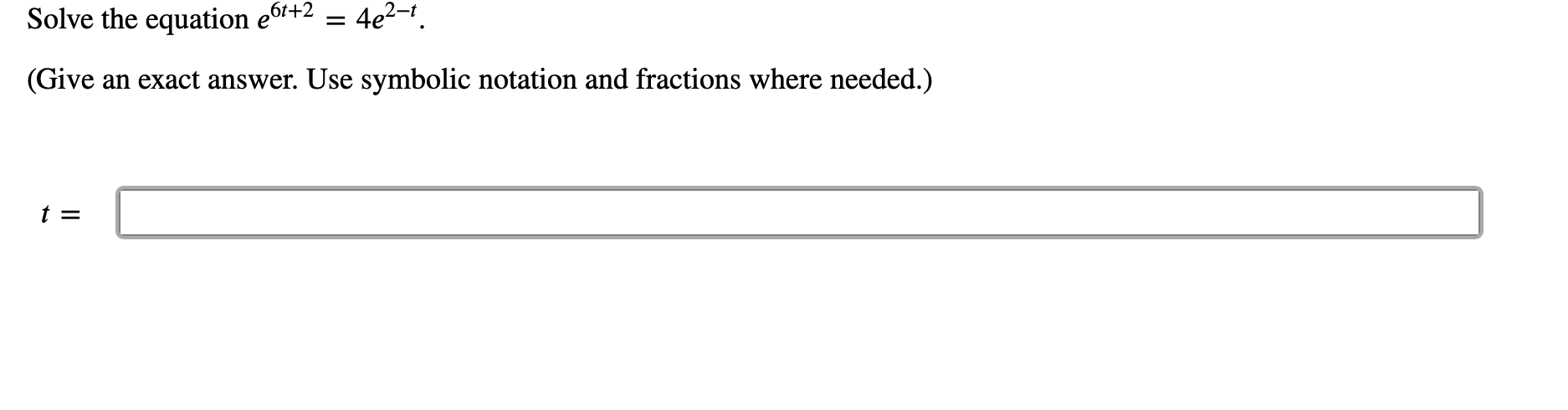# 4e2-1 Solve the equation e6'+2 (Give an exact answer. Use symbolic notation and fractions where needed.) t =

Questionhelp_outlineImage Transcriptionclose4e2-1 Solve the equation e6'+2 (Give an exact answer. Use symbolic notation and fractions where needed.) t = fullscreen